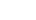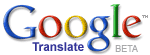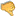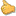ترجم الموضوع الى العربية# Sum of Square Roots for Natural Whole Numbers: A New Essay to Solve an Old Economic ProblemBrahim MANSOURI
2015 / 9 / 16

Suppose that some coalition States gain a war against a defeated country. Assume that the allied governments force the defeated State to pay a war penalty such as every year (n), the failed State has to disburse a fixed monetary sum (X) multiplied by the square root of n, with n varying from 1 to the infinite. The question to answer therefore is: What is the total amount of money the defeated State should pay at the infinite assuming that States are eternal?

This problem has received my particular attention during recent days. Even comprehensive research on the Web has not yielded expected outcomes. Many mathematical forums still discuss the issue to find a solution.

Note that I have not yet conducted deeper bibliographical research on the issue outside of the Net and I have not contacted mathematicians to get their points of view about the issue. Hence, I don�t know if a specific formula exists anywhere to solve our economic problem. Knowing that I am economist rather than mathematician, I will personally try in what follows, to find a formula to solve this thorny problem with a hope that qualified mathematicians react to our findings and, eventually, help us to improve the mathematical reasoning.

First of all, let us find a mathematical design for our economic problem. Every year (n), the defeated State should pay a fixed amount (X) multiplied by the square root of n, the latter varying from 1 to the infinity. It is easy to understand in this case that the failed State should pay at the infinite, the fixed amount (X) multiplied by the sum of square roots of all the infinite consecutive natural whole numbers (1,2,3��.,n). Formally, defining the total amount to pay and the square root of a whole number i as TAP and SQR(i) respectively, we can write:

TAP = X.SQR(1) + X.SQR(2) + X.SQR(3) +��+ X.SQR(n) = X.SUM(SQR(i)),

where SUM(SQR(i)) is the sum of square roots of i from 1 to the infinite number n.

As everyone can understand, it is very difficult for humans to compute this entire infinite sum with harder efforts to calculate and sum all the n square roots. With no computer software or, at least, a relatively powerful calculator, errors in computing and summing the square roots are seen to be unavoidable.

The best solution will be therefore to find a simple formula for the specified sum, that is SUM(SQR(i)), to be then multiplied by X to obtain the total amount to pay by the defeated State at the infinite horizon. How can this simple formula be found? In what follows, we resort to mathematical analysis (especially integrals) coupled with simple geometrical reasoning.

The starting point in our analysis is the square root ----function---- (SQR), defined in the set of real numbers (ℝ-;---;-----;-------;----), as f(x) = SQR(x), x varying from 0 (zero) to the infinity. When drawing the curve of the square root ----function----, one can understand that such a curve maps the area between the curve and the horizontal axis. To measure such area, it is easy to compute the integral of the square root --function-- between the infinite number n and 0 (zero), to obtain a value of:

area1 = 2/3.n.SQR(n).

The computed integral may be seen as an infinitesimal sum of square roots. It cannot be considered as a discrete sum of square roots we need to solve our defeated State�s economic problem. Nevertheless, the computed integral is very useful to find a good formula to calculate the discrete sum (SUM(SQR(i)) of the square roots. To do so, let us represent the n square roots as areas of the vertical rectangles breaking through the curve of the square root ----function----, with, everywhere, a value of 1 for width and SQR(i) for the length, i varying from 1 to n. Examining the curve of the square root ----function---- punctured by the various vertical rectangles, one can understand that most of the area for each rectangle is included under the curve area. In turn, the remaining area for each vertical rectangle, characterized here as error�-or-residual above the square root curve, is obviously less than the area below the curve of the square root ----function----. Therefore, the sum of the square roots (SUM(SQR(i)) for all the n natural whole numbers may be computed as the sum of the areas below and above the curve of the square root ----function----, that is the sum of the areas for all the n vertical rectangles. While in line with the integration theory, we know that the area under the square root curve is (2/3.n.SQR(n) = 2/3.SQR(n^3)), we have no ----dir----ect and clear idea about a possible measurement of the area above the curve, that is the sum of the remaining areas of the vertical rectangles. This is the major issue we deal with in the following developments in order to solve our specified economic problem.

After drawing the curve of the square root --function-- defined within the set of all positive real numbers and penciling all the vertical rectangles breaking through the drawn curve, the next intuitive idea consists of drawing another curve linking all the upper left corners of the vertical rectangles. Then, the newly drawn curve may be modeled as a --function-- g such as g(x) = SQR(x+1), with �g� being defined in the set from -1 to the infinity. Knowing that the area (area2) between the g and f curves for SQR(x+1) and SQR(x) respectively may be computed as the difference between the integrals of the two ----function----s from 0 (zero) to the infinite number n, such area will be:

AREA2 = 2/3.(( n + 1).SQR(n + 1) - n.SQR(n) - 1)

It is clear that the remaining areas of the vertical rectangles above the curve of the square root ----function---- form a certain proportion of AREA2 as formally specified through our equation above. What can be this proportion? How can mathematical reasoning help to compute it? In what extent, this proportion to be computed can be useful to find a simple formula for the sum of infinite natural whole numbers to finally solve the economic problem of the defeated State? In what follows, we try to answer these questions.

First of all, we know that the remaining areas (RA) for the vertical rectangles above the curve of the square root ----function---- (f) equals the difference between the sum of the square roots of the n infinite natural whole numbers and the integral of the f ----function---- between n and 0 (zero). Geometrically speaking, this means that such remaining areas may be measured as the difference between the sum of the areas for all the n vertical rectangles and the area below the square root curve until the horizontal axis. Formally, we can write:

RA = SUM(SQR(i)) � 2/3.n.SQR(n)

We argue that at an infinite horizon, the proportion of the whole subarea (RA) to AREA2 will be:

RA/AREA2 = (SUM(SQR(i)) � 2/3.n.SQR(n))/(2/3.((n+1).SQR(n+1) - n.SQR(n))) = 1/2

This formula for the proportion of RA to AREA2 may be then used to compute the required sum of the square roots of the n natural whole numbers. The idea is straightforward: the required sum equals the area under the square root ----function---- (f), that is the area noted AREA1, to be augmented by adding the half of the area between the g and f curves, that is the half of the area noted AREA2. Formally, we can write for i varying from 0 (zero) to n:

SUM(SQR(i)) = AREA1 + 0.50.AREA2

This means that:

SUM(SQR(i)) = 2/3.n.SQR(n) + 0.50.(2/3.((n+1).SQR(n+1) - n.SQR(n) - 1))
= 1/3.((n+1).SQR(n+1) +n.SQR(n) - 1)
= 1/3.(SQR((n+1)^3) + SQR(n^3) - 1)

Therefore, for an infinite n number, to obtain the sum of the square roots of the n natural whole digits, we should add the third of the square root for the (n+1)th number powered to 3, that is SQR((n+1)^3), to the third of the nth number powered to 3, that is SQR(n^3), and then subtract one third from such sum.

The formula seems to perform well even for smaller values of n. Taking examples, we find that:

- for the 10 first natural whole numbers, the estimated sum of the square roots according to our formula, is about 19.21 against a very close value of nearly 19.30 for the effective sum as computed using a computer software----;----
- for the 20 first numbers, the estimated sum is about 19.09 against an effective value of 19.19----;----
- for the 100 first natural numbers, estimates yield 661.35 compared to an effectively computed value of 661.46----;----
- the estimated square roots� sum for the first 1000 natural whole numbers is 21065.72 against a real-world value of 21065.83.

As soon as n grows, the gap between the estimated and effective values of the square roots� sum evanishes. For instance, when n equals 1000000, the estimated and effective square roots� sums are seen to be identical, with a same value of about 667000000. For n = 4000000, the estimated and effective square roots� sums have the same value of nearly 5330000000.

With respect to the defeated State�s economic problem as above specified, our estimates reveal that this State should pay at a time horizon of n years, a war penalty (WP) of about:

WP = X.(1/3.(SQR((n + 1)^3) + SQR(n^3) - 1))

Even for shorter time horizons, our estimates reveal that the found formula performs well to forecast the amount of war penalty in accordance with the initially specified economic problem. We know that, in our modern era with developed technologies, it is easier, through computer software packages, to compute the sum of square roots as well as to undertake other complex operations, but the designed formula may be useful, at least methodologically. It may also be used to solve other economic and extra-economic problems. Comments and suggestions are welcome�

By Dr. Brahim MANSOURI, Professor of economics, Faculty of Juridical, Economic and Social Sciences, Cadi Ayyad University, Marrakech, Morocco. Marrakech, September, 15th, 2015.Bad 12345678910 Very good